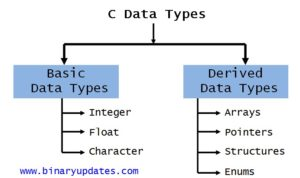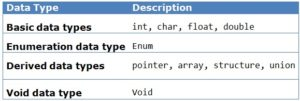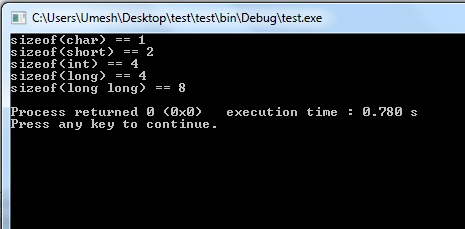Monday , May 20 2019

# Variables and Data Types in C Programming

Let’s learn about variables and data types in C Programming. We will first look at Variables in C; Variables are used to store the value during the execution of a program. The name itself means, the value of variable can be changed hence the name “Variable“. The variables are stored in Main Memory i.e. RAM (size depending on the data type). In C Programming we always have to declare variable before we can use them. Note that the space is allocated to variable in memory during execution or run-time.

C is a strongly typed language. What this means it that, the type of a variable cannot be changed. Suppose we declared an integer type variable so we cannot store character or a decimal number in that variable.

There are set of rules to be followed while declaring variables and data types in C Programming:

• The 1st letter should be alphabet.
• Variables can be combination of alphabets and digits.
• Underscore (_) is the only special character allowed.
• Variables can be written in both Uppercase and Lowercase or combination of both.
• Variables are Case Sensitive.
• No Spaces allowed between Characters.
• Variable name should not make use to the C Reserved Keywords.
• Variable name should not start with a number.Variables and Data Types in CData Types in C Programming Language

#### EXAMPLE 1: Integer Variable in C

The %d is to tell printf() function to format the integer i as a decimal number. The output from this program would be This is my integer: 10.

#### EXAMPLE 2: Float Variable in C

The %f is to tell printf() function to format the float variable f as a decimal floating number. The output from this program would be This is my float: 3.1415. Now if we want to see only the first 2 numbers after the floating point, we would have to modify printf() function call to be as given below:
After replacing printf function in a given example. The output from this program would be This is my float: 3.14.

#### EXAMPLE 3: Character Variable in C

The %c is to tell printf() function to format the variable “c” as a character. The output from this program would be This is my character: b.

#### Data types in C Programming

All the data types defined by C are made up of units of memory called bytes. On most computer architectures a byte is made up of eight bits, each bit stores a one or a zero. These eight bits with two states give 256 combinations (28). So an integer which takes up four bytes can store a number between 0 to 4,294,967,295 (0 and 232). However, integer variables use the first bit to store whether the number is positive or negative so their value will be between -2,147,483,648 and + 2,147,483,647.

As we mentioned, there are eight basic data types defined in the C language. Five types for storing integers of varying sizes and three types for storing floating point values (values with a decimal point). C doesn’t provide a basic data type for text. Text is made up of individual characters and characters are represented by numbers. In the last example we used one of the integer types: int. This is the most commonly used type in the C language.

 Data Type Size Value Range char 1 byte -128 to 127 or 0 to 255 unsigned char 1 byte 0 to 255 signed char 1 byte -128 to 127 int 2 or 4 bytes -32,768 to 32,767 or -2,147,483,648 to 2,147,483,647 unsigned int 2 or 4 bytes 0 to 65,535 or 0 to 4,294,967,295 short 2 byte -32,768 to 32,767 unsigned short 2 byte 0 to 65,535 long 4 byte -2,147,483,648 to 2,147,483,647 unsigned long 4 byte 0 to 4,294,967,295

The char data type is usually one byte, it is so called because they are commonly used to store single characters. The size of the other types is dependent on machine. Most desktop machines are “32-bit”, this refers to the size of data that they are designed for processing. On “32-bit” machines the int data type takes up 4 bytes (232). The short is usually smaller, the long can be larger or the same size as an int and finally the long long is for handling very large numbers.

The type of variable you use generally doesn’t have a big impact on the speed or memory usage of your application. Unless you have a special need you can just use int variables. We will try to point out the few different cases like. A decade ago, most machines had 16-bit processors, this limited the size of int variables to 2 bytes. At the time, short variables were usually also 2 bytes and long would be 4 bytes. Nowadays, with 32-bit machines, the default type (int) is usually large enough to satisfy what used to require a variable of type long. The long long type was introduced more recently to handle very large numeric values.

#### EXAMPLE 3: Calculate Size of Data type on Your Machine

To find out the size of each data type on your machine compile and run this program. It uses one new language construct sizeof(). This tells us how many bytes a data type takes up.

The output from this program would be:Output of Sizeof Data Types

Some computers are better at handling really big numbers, so the size of the data types will be bigger on these machines. Now we know most basic building blocks i.e. variables and data types in C Programming. In next lesson we will covers Operators in C Programming which allows us to solve many computing issues while programming.

### About Umesh LokhandeUmesh Lokhande holds a Master degree in Scientific Instrumentation from University of Applied Sciences Jena, Germany. and has previously worked at Orbotech, Alere Technologies etc. Umesh is also a founder and first author of BINARYUPDATES.COM